How Cheenta works to ensure student success?
Explore the Back-Story

# Test of Mathematics Solution Subjective 49 - Arrangement of Similar ItemsThis is a Test of Mathematics Solution Subjective 49 (from ISI Entrance). The book, Test of Mathematics at 10+2 Level is Published by East West Press. This problem book is indispensable for the preparation of I.S.I. B.Stat and B.Math Entrance.

Also visit: I.S.I. & C.M.I. Entrance Course of Cheenta

## Problem: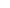red balls,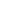black balls,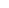white balls are to be arranged in a row. Suppose that any two balls of the same color are indistinguishable. Given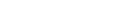prove that number of possible arrangements is maximum when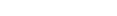.

## Solution:

Given a total of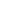objects out of which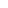are of the same type, total number of arrangements possible is given by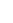. Therefore in this case the total number of arrangements is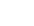.

Now we have to maximise.

Thus we need to minimise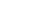. As the question claims that all have to be equal to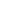, let us consider that they are not all equal to. So the cases that arise are:

Case 1: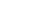,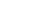,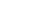OR

Case 2:,,OR

Case 3:,,These cases are considered without any loss of generality as any other possible case will just be another permutation of the given cases.

So as the question claims the ideal condition is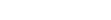.

Now considering Case 1, as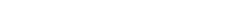=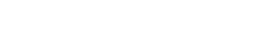. So this is not a possible option as we have to minimise.

Now taking Case 2,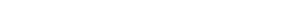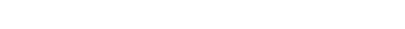. So again not a possibility.

Similarly it can be shown for Case 3 also, thus proving that any other value of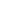anddoes not minimise.

Henceis the solution that maximises the number of possible arrangements.This is a Test of Mathematics Solution Subjective 49 (from ISI Entrance). The book, Test of Mathematics at 10+2 Level is Published by East West Press. This problem book is indispensable for the preparation of I.S.I. B.Stat and B.Math Entrance.

Also visit: I.S.I. & C.M.I. Entrance Course of Cheenta

## Problem:red balls,black balls,white balls are to be arranged in a row. Suppose that any two balls of the same color are indistinguishable. Givenprove that number of possible arrangements is maximum when.

## Solution:

Given a total ofobjects out of whichare of the same type, total number of arrangements possible is given by. Therefore in this case the total number of arrangements is.

Now we have to maximise.

Thus we need to minimise. As the question claims that all have to be equal to, let us consider that they are not all equal to. So the cases that arise are:

Case 1:,,OR

Case 2:,,OR

Case 3:,,These cases are considered without any loss of generality as any other possible case will just be another permutation of the given cases.

So as the question claims the ideal condition is.

Now considering Case 1, as=. So this is not a possible option as we have to minimise.

Now taking Case 2,. So again not a possibility.

Similarly it can be shown for Case 3 also, thus proving that any other value ofanddoes not minimise.

Henceis the solution that maximises the number of possible arrangements.

### Knowledge Partner